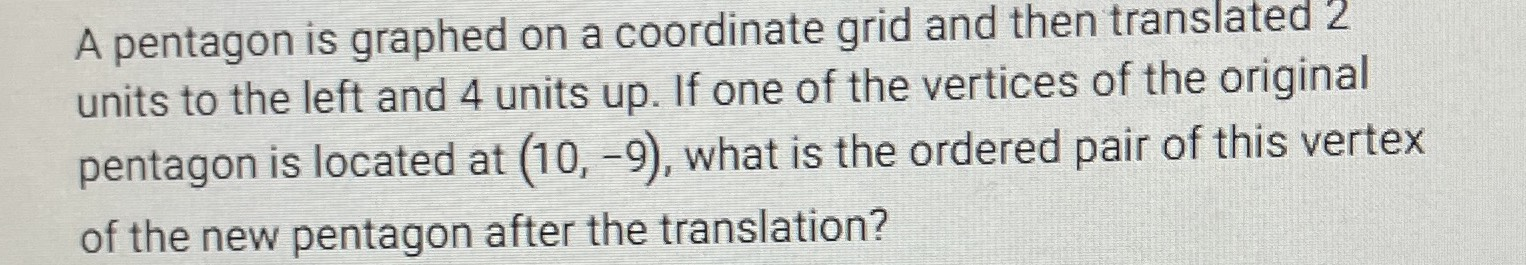### ¿Todavía tienes preguntas de matemáticas?

Pregunte a nuestros tutores expertos
Algebra
PreguntaA pentagon is graphed on a coordinate grid and then translated $$2$$ units to the left and $$4$$ units up. If one of the vertices of the original pentagon is located at $$( 10 , - 9 ) ,$$ what is the ordered pair of this vertex of the new pentagon after the translation?